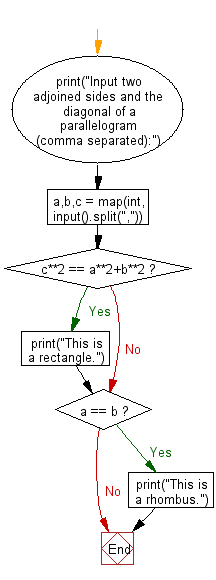﻿ Python: Reads the two adjoined sides and the diagonal of a parallelogram and check whether the parallelogram is a rectangle or a rhombus - w3resource# Python: Reads the two adjoined sides and the diagonal of a parallelogram and check whether the parallelogram is a rectangle or a rhombus

## Python Basic - 1: Exercise-49 with Solution

Write a Python program which reads the two adjoined sides and the diagonal of a parallelogram and check whether the parallelogram is a rectangle or a rhombus.

According to Wikipedia-
parallelograms: In Euclidean geometry, a parallelogram is a simple (non-self-intersecting) quadrilateral with two pairs of parallel sides. The opposite or facing sides of a parallelogram are of equal length and the opposite angles of a parallelogram are of equal measure.
rectangles: In Euclidean plane geometry, a rectangle is a quadrilateral with four right angles. It can also be defined as an equiangular quadrilateral, since equiangular means that all of its angles are equal (360°/4 = 90°). It can also be defined as a parallelogram containing a right angle.
rhombus: In plane Euclidean geometry, a rhombus (plural rhombi or rhombuses) is a simple (non-self-intersecting) quadrilateral whose four sides all have the same length. Another name is equilateral quadrilateral, since equilateral means that all of its sides are equal in length. The rhombus is often called a diamond, after the diamonds suit in playing cards which resembles the projection of an octahedral diamond, or a lozenge, though the former sometimes refers specifically to a rhombus with a 60° angle, and the latter sometimes refers specifically to a rhombus with a 45° angle.

Input:
Two adjoined sides and the diagonal.
1 ≤ ai, bi, ci ≤ 1000, ai + bi > ci
Input two adjoined sides and the diagonal of a parallelogram (comma separated):
3,4,5
This is a rectangle

Sample Solution:

Python Code:

``````print("Input two adjoined sides and the diagonal of a parallelogram (comma separated):")
a,b,c = map(int, input().split(","))
if c**2 == a**2+b**2 :
print("This is a rectangle.")
if a == b:
print("This is a rhombus.")
``````

Sample Output:

```Input two adjoined sides and the diagonal of a parallelogram (comma separated):
3,4,5
This is a rectangle.```

Pictorial Presentation:Flowchart:Python Code Editor:

Have another way to solve this solution? Contribute your code (and comments) through Disqus.

What is the difficulty level of this exercise?

Test your Programming skills with w3resource's quiz.

﻿

## Python: Tips of the Day

Iterating over dictionaries using 'for' loops:

I am a bit puzzled by the following code: d = {'x': 1, 'y': 2, 'z': 3} for key in d: print key, 'corresponds to', d[key] What I don't understand is the key portion. How does Python recognize ...

key is just a variable name.

```for key in d:
```

For Python 3.x:

```for key, value in d.items():
```

For Python 2.x:

```for key, value in d.iteritems():
```

To test for yourself, change the word key to poop.

In Python 3.x, iteritems() was replaced with simply items(), which returns a set-like view backed by the dict, like iteritems() but even better. This is also available in 2.7 as viewitems().

The operation items() will work for both 2 and 3, but in 2 it will return a list of the dictionary's (key, value) pairs, which will not reflect changes to the dict that happen after the items() call. If you want the 2.x behavior in 3.x, you can call list(d.items()).

Ref: https://bit.ly/37dm0Qo